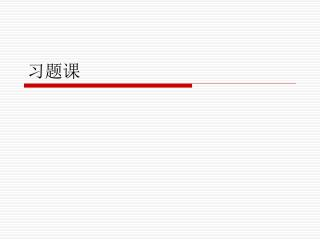DownloadDownload Presentation习题课

Download Presentation习题课

- - - - - - - - - - - - - - - - - - - - - - - - - - - E N D - - - - - - - - - - - - - - - - - - - - - - - - - - -
Presentation Transcript

1. 习题课

2. 7.6 • The following sequence of virtual page numbers is encountered in the course of execution on a computer with virtual memory: 3 4 2 6 4 7 1 3 2 6 3 5 1 2 3 Assume that a least recently used page replacement is adopted. Plot a graph of page hit radio (fraction of page references in which the page is in main memory) as a function of main memory page capacity n for 1≤n≤8 . And assume that main memory is initially empty.

3. 7.6 Answer: Hit ratio =0 Hit ratio=2÷15×100%≈13.3 %

4. 7.6 Answer: Hit ratio=2÷15×100%≈13.3 % 4.n=4 Hit ratio =3÷15×100 %=20 %

5. 7.6 Answer: Hit ratio =5÷15×100 % =33.3 %

6. 7.6 Answer: Hit ratio =8÷15×100 %=53.3 %

7. 7.6 Answer: Hit ratio =8÷15×100 %=53.3 %

8. 7.6 Answer: Hit ratio =8÷15×100 %=53.3 %

9. 7.6 Answer: • The graph of the hit ratio is:

12. 8.15 Answer: • a. -5=-101B=-0.101*2^3 E=127+3=10000010B S=1.01 Sign bit Biased exponent Significand 1 10000010 01000000000000000000000 • b. -6=-110B=-0.110*2^3 E=127+3=10000010B S=1.10 Sign bit Biased exponent Significand 1 10000010 10000000000000000000000

13. 8.15 Answer: • c. -1.5=-1.1B=-0.11*2 E=127+1=10000000B S=1.1 Sign bit Biased exponent Significand 1 10000000 10000000000000000000000 • d. 384=110000000B=0.11*2^9 E=127+9=10001000B S=1.1 Sign bit Biased exponent Significand 0 10001000 10000000000000000000000

14. 8.15 Answer: • e. 1/16=0.0001B=0.1*2^(-3) E=127-3=1111100B S=1.0 Sign bit Biased exponent Significand 0 01111100 00000000000000000000000 • f. -1/32=-0.00001B=-0.1*2^(-4) E=127-4=1111011B S=1.0 Sign bit Biased exponent Significand 1 01111011 00000000000000000000000

15. 11.3Answer: • equation 11.1 • Equation 11.2

16. 15.4 • To reduce control field width from 11 to 8, we should divide 11 control signals into 3 fields, each field contains 3 control signals that are mutually exclusive, so we use 2 bits encoding control to replace 3 bits direct control, residue 2 bit is direct control. • Key to solve the question is to find 3 fields and each field contain 3 control signal mutually exclusive. We discover that C4 and C7 must be direct controlling signals;

17. 15.4 Answer: • to find mutually exclusive control signal: 1 2 3 5 6 8 9 10 111 3 6 8 9 1 3 9 2 5 6 8 10 112 5 6 8 2 5 8 6 10 11 So the result is: 01 C1 01 C2 01 C6 10 C3 10 C5 10 C10 11 C9 11 C8 11 C11 C4 C7